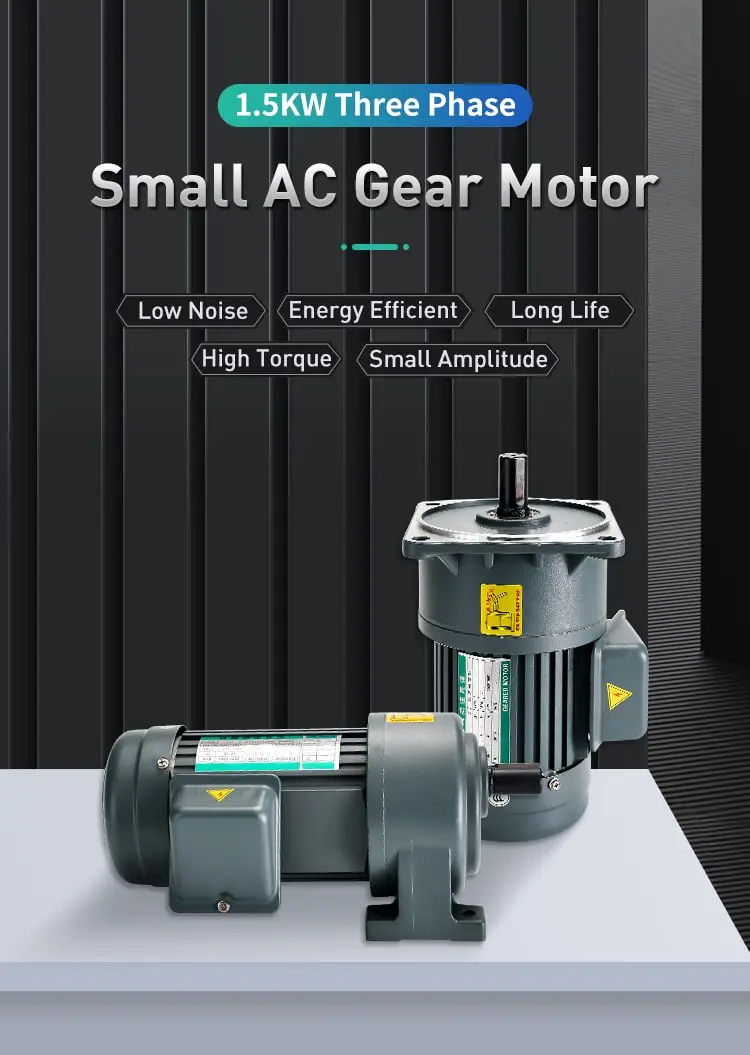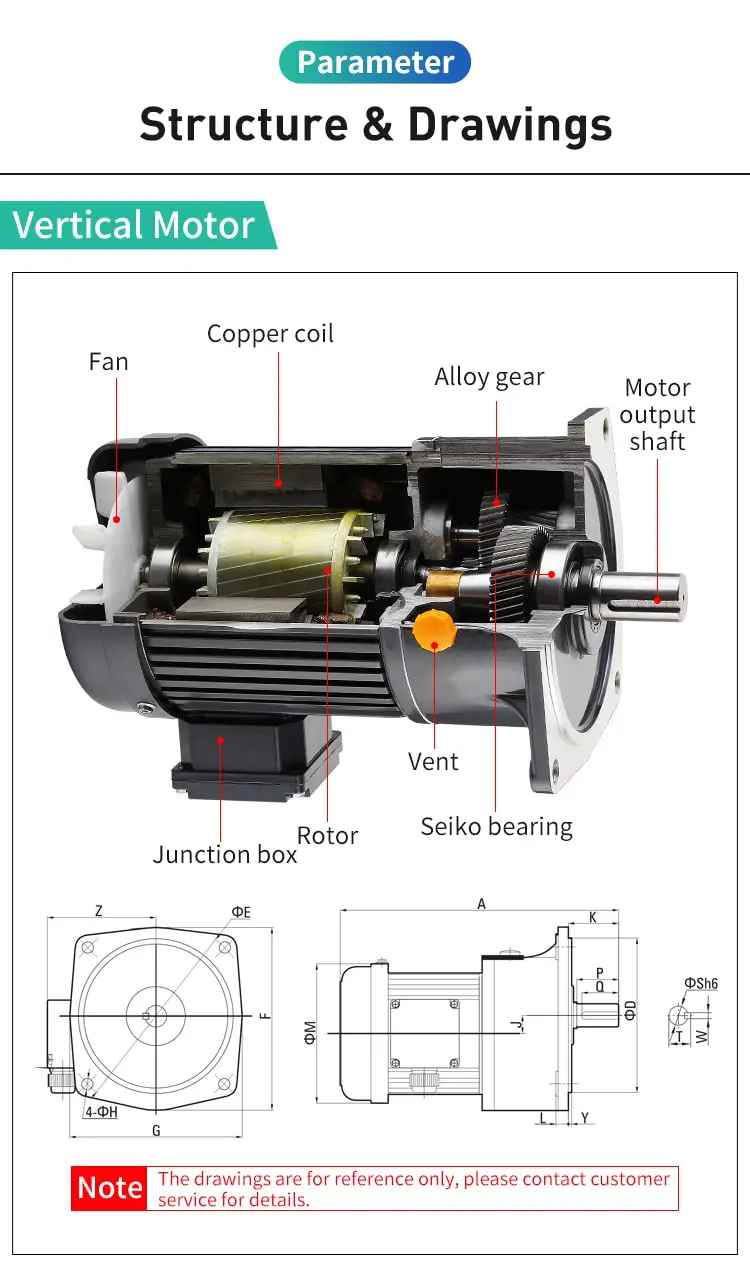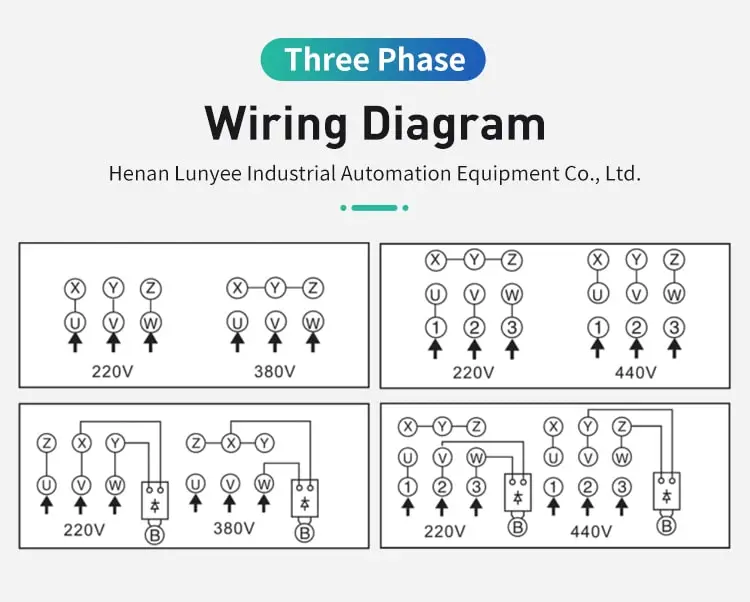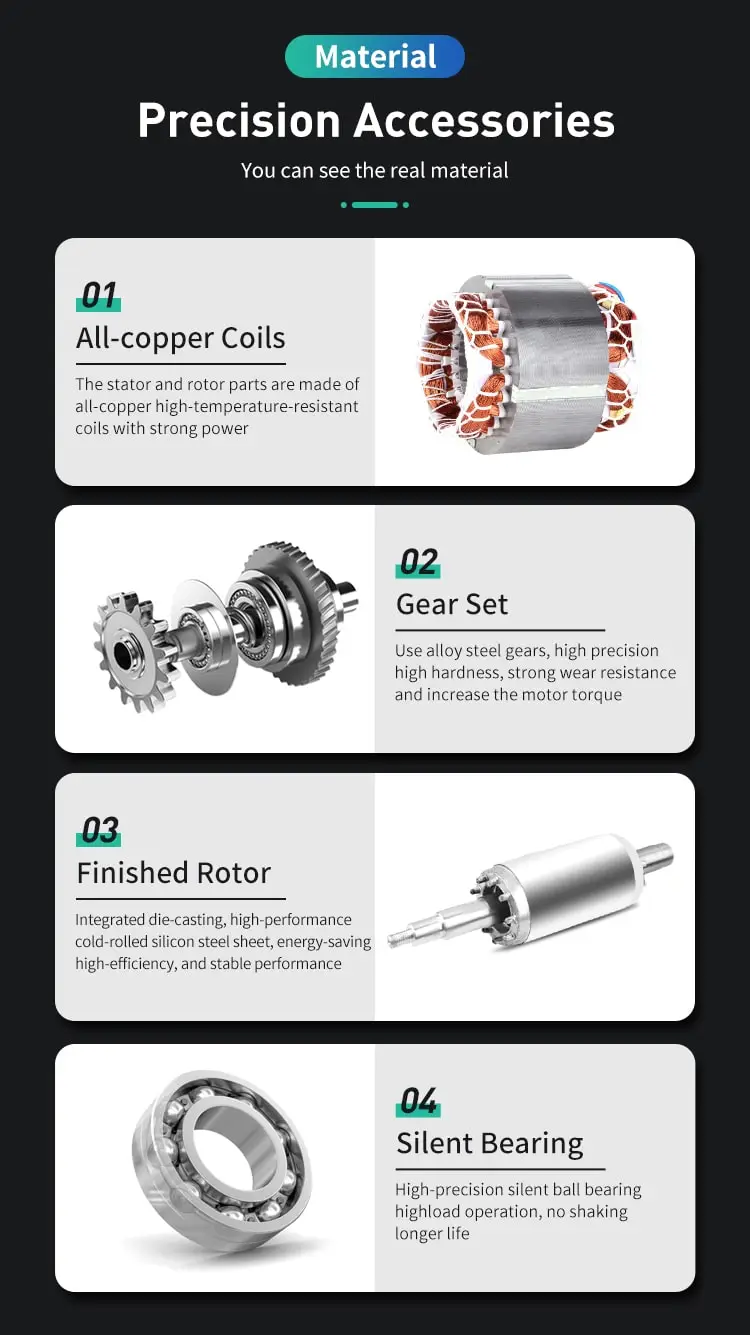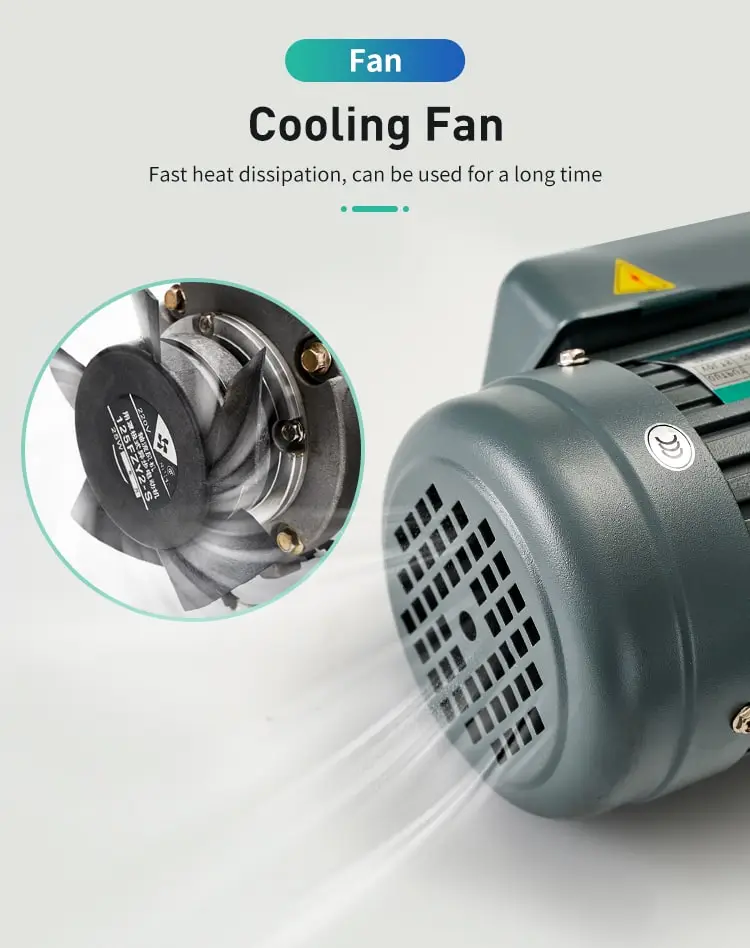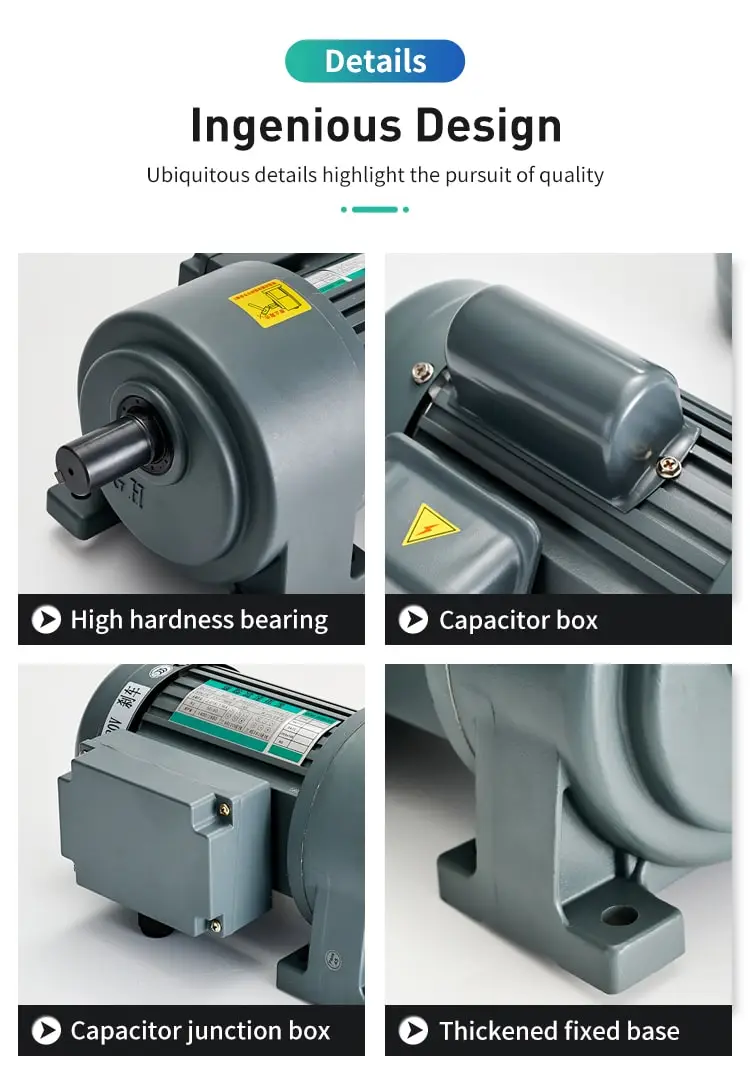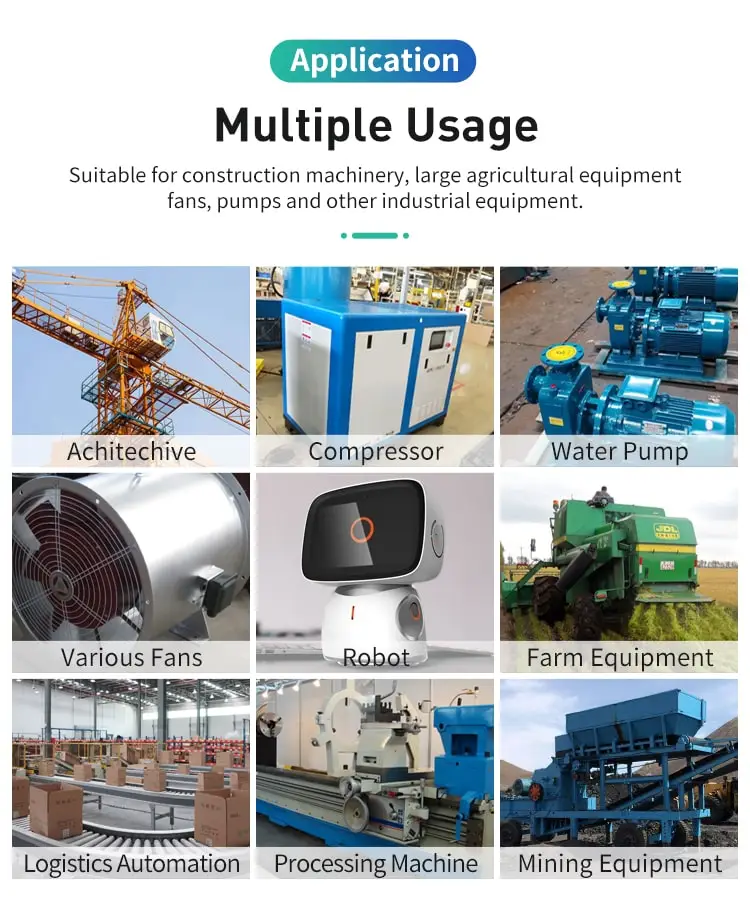#### How To Test DC Motor?

Testing a DC motor is an important step in ensuring that it is functioning properly and is capable of providing the desired performance and reliability. In this

Testing a DC motor is an important step in ensuring that it is functioning properly and is capable of providing the desired performance and reliability. In this essay, we will explore the various steps and considerations involved in testing a DC motor, including the following:

1. Preparing the test equipment and tools

2. Measuring the DC motor voltage and current

3. Testing the DC motor speed and torque

4. Evaluating the DC motor performance and efficiency

5. Preparing the test equipment and tools

Before testing a DC motor, it is important to gather the necessary equipment and tools. This may include a multimeter, an oscilloscope, a load tester, and a dynamometer.

A multimeter is a versatile instrument that is used to measure various electrical parameters, including voltage, current, resistance, and continuity. A multimeter is essential for testing a DC motor, as it can be used to measure the voltage and current supplied to the motor.

An oscilloscope is a specialized instrument that is used to measure and analyze electronic signals, such as voltage and current. An oscilloscope is useful for testing a DC motor, as it can be used to measure the waveform of the voltage and current supplied to the motor, as well as the speed and torque of the motor.

A load tester is a device that is used to apply a load to the DC motor, simulating the actual operating conditions of the motor. A load tester is essential for testing the speed and torque of the DC motor, as it allows for accurate and repeatable measurements.

A dynamometer is a device that is used to measure the torque and power output of the DC motor. A dynamometer is useful for evaluating the performance and efficiency of the DC motor, as it allows for precise and accurate measurements of the motor's output.

1. Measuring the DC motor voltage and current

Once the test equipment and tools have been prepared, the next step is to measure the voltage and current supplied to the DC Motor . This can be done using a multimeter, which should be connected to the power supply and the DC motor as shown in the diagram below:

[Diagram of a DC motor connected to a multimeter]

To measure the voltage and current supplied to the DC motor, the multimeter should be set to the appropriate range and mode. For example, if the DC motor is supplied with 12 volts, the multimeter should be set to the 20 volt DC range.

Once the multimeter is set up and connected, the voltage and current supplied to the DC motor can be measured. The voltage should be measured across the power supply terminals, and the current should be measured through the DC motor terminals.

The measured voltage and current should be compared to the specifications of the DC motor to ensure that they are within the acceptable range. If the measured values are outside the acceptable range, it may indicate a problem with the power supply or the DC motor, and further testing may be required.

1. Testing the DC motor speed and torque

After the voltage and current supplied to the DC motor have been measured, the next step is to test the speed and torque of the DC motor. This can be done using an oscilloscope, a load tester, and a dynamometer.

To test the speed and torque of the DC motor, the oscilloscope should be connected to the DC motor as shown in the diagram below:

[Diagram of a DC motor connected to an oscilloscope]

The oscilloscope should be set to the appropriate range and mode, and the trigger should be set to capture the waveform of the voltage and current supplied to the DC motor.

Once the oscilloscope is set up and connected, the speed and torque of the DC motor can be tested. The oscilloscope can be used to measure the waveform of the voltage and current supplied to the motor, which can be used to calculate the speed and torque of the motor.

To measure the speed of the DC motor, the oscilloscope can be used to measure the frequency of the voltage and current waveform. The speed of the DC motor is directly proportional to the frequency of the waveform, and it can be calculated using the following formula:

Speed (RPM) = (Frequency (Hz) * 60) / Number of poles

To measure the torque of the DC motor, the load tester and the dynamometer can be used to apply a load to the motor and measure the torque output. The load tester can be used to apply a load to the motor, simulating the actual operating conditions of the motor. The dynamometer can then be used to measure the torque output of the motor, which can be calculated using the following formula:

Torque (Nm) = (Power (W) * 9.5493) / Speed (RPM)

The measured speed and torque of the DC motor should be compared to the specifications of the motor to ensure that they are within the acceptable range. If the measured values are outside the acceptable range, it may indicate a problem with the DC motor, and further testing may be required.

1. Evaluating the DC motor performance and efficiency

After the speed and torque of the DC motor have been measured, the next step is to evaluate the performance and efficiency of the DC motor. This can be done by measuring the input and output power of the DC motor, and calculating the efficiency using the following formula:

Efficiency (%) = (Output Power (W) / Input Power (W)) * 100

To measure the input and output power of the DC motor, the multimeter and the dynamometer can be used. The multimeter can be used to measure the voltage and current supplied to the DC motor, and the dynamometer can be used to measure the torque and speed of the motor. The input and output power can then be calculated using the following formulas:

Input Power (W) = Voltage (V) * Current (A) Output Power (W) = Torque (Nm) * Speed (RPM) * 0.1047

The measured efficiency of the DC motor should be compared to the specifications of the Lunyee Motor to ensure that it is within the acceptable range. If the measured efficiency is outside the acceptable range, it may indicate a problem with the DC motor, and further testing may be required.

In conclusion, testing a DC motor involves several steps and considerations, including preparing the test equipment and tools, measuring the voltage and current, testing the speed and torque, and evaluating the performance and efficiency. By following these steps and using the appropriate equipment and formulas, the performance and reliability of a DC motor can be accurately and reliably tested.